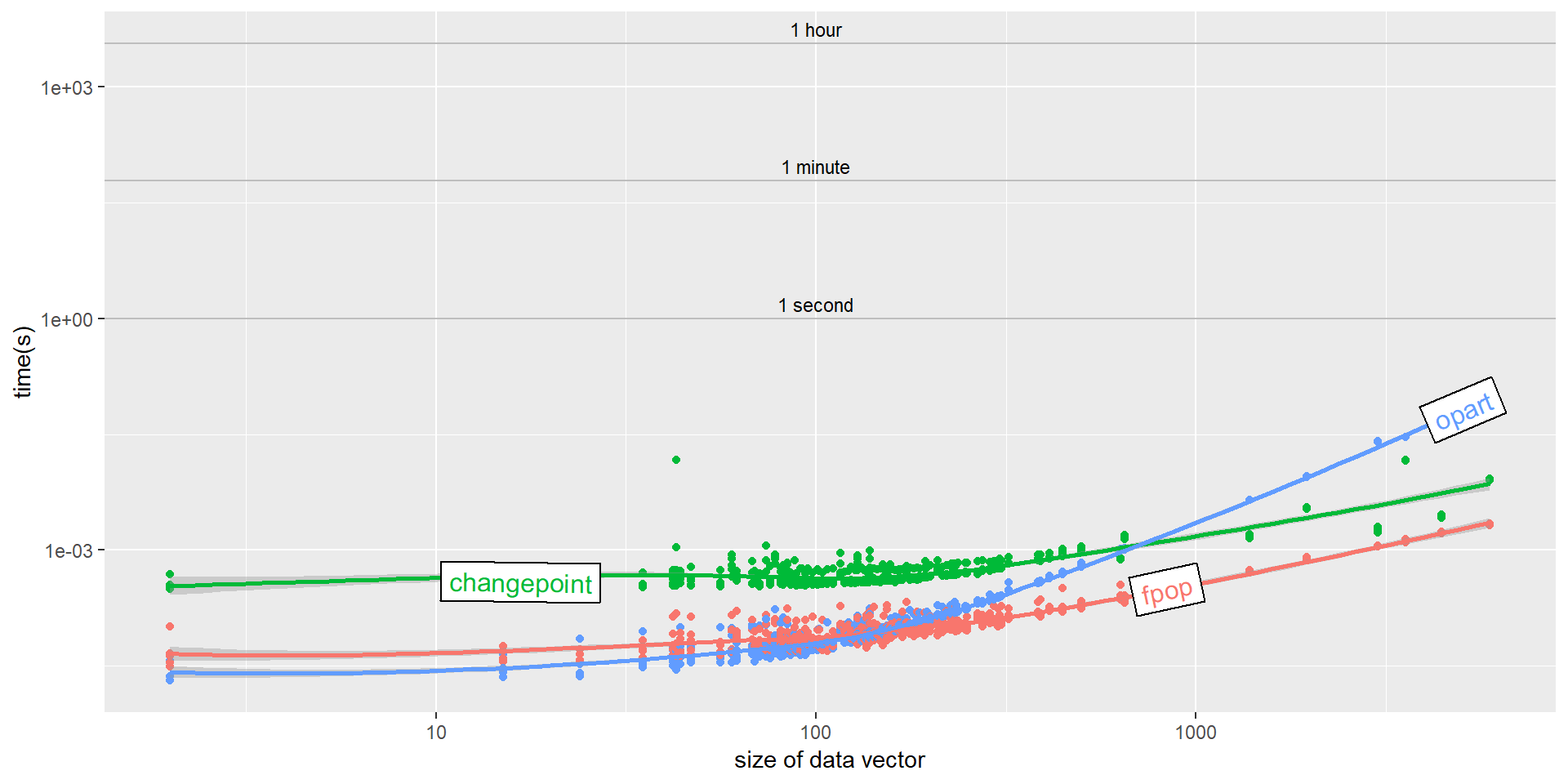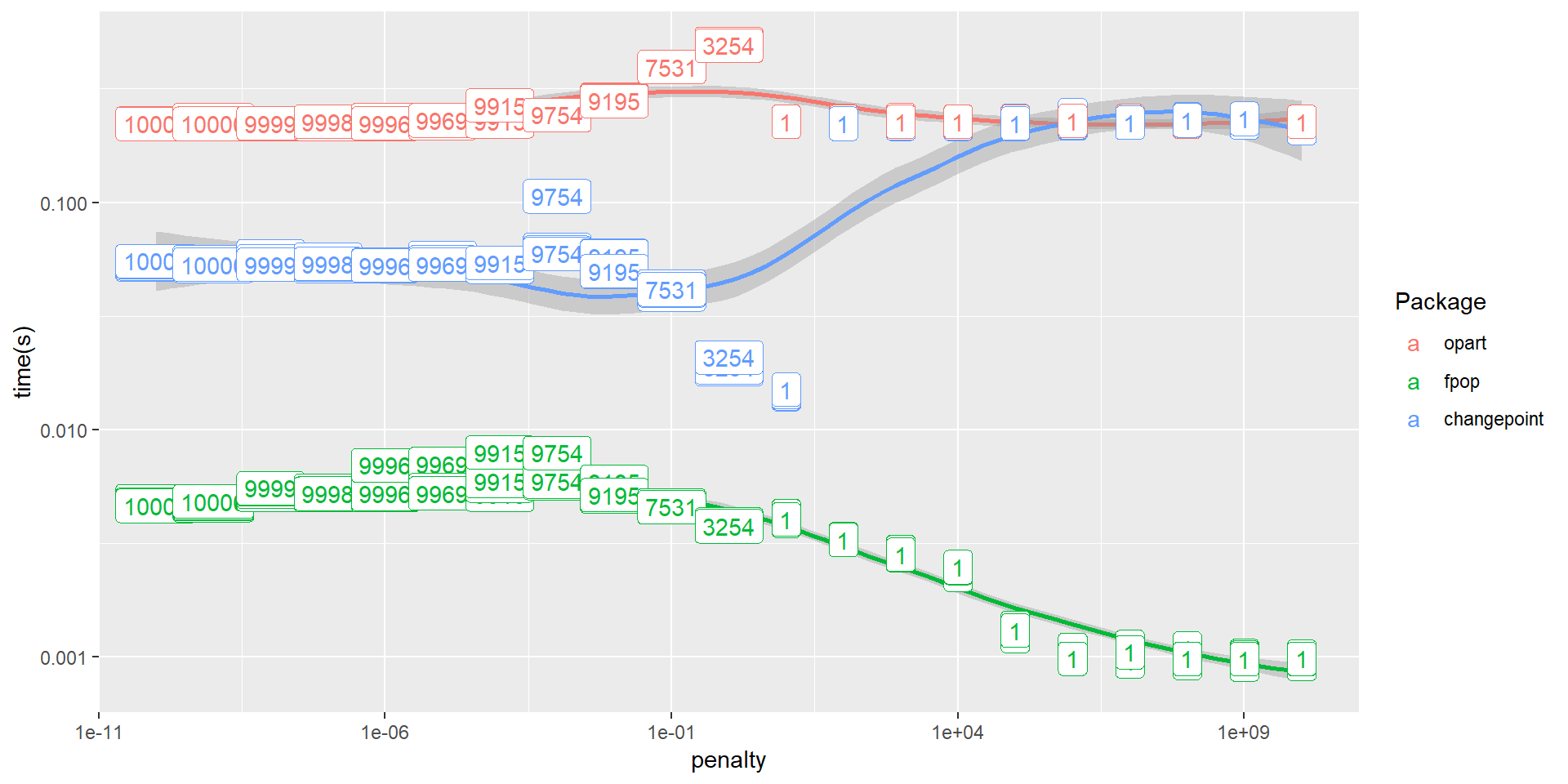### Optimal Partitioning

The content discussed in this vignette is inspired from fpop paper (by Toby Hocking, Guillem Rigaill, Paul Fearnhead). This vignette summarizes the mathematics behind optimal partitioning algorithm in statistics using square error loss / gaussian likelihood to maximize function and functions available in this package for solving the standard optimal partitioning problem using square error loss.

### Introduction

There are several applications where we need to work with ordered data (e.g. Time-series, Financial data, climate data, bioinformatics etc.). This kind of data often experiences abrupt changes in structure known as changepoints or breakpoints. It is important to detect these changepoints or breakpoints in order to model the data effectively.

There are wide-range of approaches for detecting changepoints. The optimal partitioning algorithm implemented in this package belongs to class of approaches for detecting changepoints that can be formulated in terms of defining a cost function for segmentation. The optimal segments are can then be found by either minimising a penalised version of this cost (e.g. Yao 1988; Lee 1995), which we call the penalised minimisation problem; or minimise the cost under a constraint on the number of changepoints(e.g. Yao and Au 1989; Braun and Muller 1998), which we call the constrained minimisation problem.

If the cost function depends on the data through a sum of segment-specific costs then the minimisation can be done exactly using dynamic programming (Auger and Lawrence 1989; Jackson et al. 2005) which is of atleast quadratic time complexity. The optimal partitioning algorithm implemented in this package uses this approach as our goal here is to provide an efficient C/C++ reference implementation to the standard Optimal Partitioning algorithm which can be modfied easily to develop other changepoint models.

We can understand the idea behind optimal partitioning as follows:

Let y = $$(y_{1},...,y_{n})$$ denote the data segment. Then for $$t \ge s$$, the set of observations from time s to t is denoted as $$y_{s:t} = (y_{s},..,y_{t})$$.

Now, consider segmenting the data $$y_{1:t}$$. Denote F(t) to be the minimum value of the penalised cost for segmenting such data and $$\beta$$ be the penalty due to changepoint, with F(0)=$$−\beta$$ . The idea of Optimal Partitioning is to split the minimisation over segmentations into the minimisation over the position of the last changepoint, and then the minimisation over the earlier changepoints. We can then use the fact that the minimisation over the earlier changepoints will give us the value F($$\tau^*$$) for some $$\tau^*$$ < t.

Then we have following:

$F(t) = \min_{\tau, k}\sum_{j=0}^{k}[C(y_{\tau_j + 1: \tau_{j+1}}) + \beta] - \beta$

where ‘C’ denotes the cost function which is square error loss in this case

$F(t) = \min_{\tau, k}\sum_{j=0}^{k - 1}[C(y_{\tau_j + 1: \tau_{j+1}}) + C(y_{\tau_k + 1}: t) + \beta] - \beta$

$=>F(t)= \min_{\tau^{*}}(\min_{\tau, k^{'}}\sum_{j=0}^{k^{'}}[C(y_{\tau_j + 1: \tau_{j+1}}) + \beta] - \beta + C(y_{\tau^*+1:t}) + \beta)$

$=>F(t)= \min_{\tau^*}(F(\tau^*) + C(y_{\tau^*+1:t}) + \beta)$

Hence, we obtain a simple recursion for the F(t) values

$F(t) = \min_{0 \le \tau < t}[F(t) + C(y_{\tau+1:t}) + \beta]$

The segmentations themselves can be recovered by first taking the arguments which minimise this equation.

$\tau^{*}_{t}=\min_{0≤\tau<t}[F(\tau)+C(y_{\tau+1:t})+\beta]$

which give the optimal location of the last changepoint in the segmentation of $$y_{1:t}$$.

If we denote the vector of ordered changepoints in the optimal segmentation of $$y_{1:t}$$ by cp(t), with $$cp(0)=\phi$$ , then the optimal changepoints up to a time t can be calculated recursively.

$cp(t)=(cp(\tau^{*}_{t}),\tau^{*}_{t})$

As F(t) is calculated for time steps t = 1,2,…,n and each time step involves a minimisation over $$\tau$$ = 0,1,…,t−1 the computation takes O($$n^2$$) time.

### Programming with opart

This package provides following function for optimal partitioning using square error loss:

opart_gaussian: This function computes the optimal changepoint model for a vector of real-valued data and a non-negative real-valued penalty, given the square loss (to minimize) / gaussian likelihood (to maximize).

usage: opart_gaussian(data.vec, penalty)

data.vec is the data vector on which optimal partitioning is to be performed.

penalty is any finite positive real valued number

The output has following components:

• cost.vec is a vector of optimal cost values of the best models from 1 to n_data.

• end.vec is a vector of optimal segment ends

Using neuroblastoma dataset in which normalized copy number profiles are available as profiles data frame and the breakpoint annotations are available as annotations data frame from patients with Tumors at the Institut Curie.

profiles$logratio is the normalized logratio of the probe which is proportional to copy number which we will use to find optimal segments. The following code shows the basic usage on neuroblastoma data set with log ratio values of a patient with profile id = 1 for one chromosome as data.vec and penalty equals 1. data(neuroblastoma, package="neuroblastoma") selectedData <- subset(neuroblastoma$profiles, profile.id=="1" & chromosome=="1")
result <- opart::opart_gaussian(selectedData$logratio, 1) str(result) #> List of 2 #>$ cost.vec: num [1:474] -0.201 -0.414 -0.664 -0.988 -1.211 ...
#>  $end.vec : int [1:4] 187 437 460 474 ### Model comparison with fpop and cpt.mean Here we compare the model produced by opart for different profile ids in neuroblastoma data set and compare it to the model produced with fpop and cpt.mean. Since all the 3 algorithms use the same cost function we should get same segment ends. In other words the “end.vec” produced by opart should match with the corresponding vector of segment ends in fpop and cpt.mean. We also vary penalty in each iteration. n.profiles <- 10 selected_ids <- unique(neuroblastoma$profiles$profile.id) profile_ids_vec <- head(selected_ids, 10) selected_data <- neuroblastoma$profiles

#initializing lists for storing the results of 3 algorithms
fpop_res <- list()
opart_res <- list()
cpt_res <- list()

#counting the iterations
iters <- 0

for(profile_id in profile_ids_vec){
current_data = subset(selected_data, profile.id == profile_id)

fpop_res <- fpop::Fpop(current_data$logratio, iters + 1) cpt_res <- changepoint::cpt.mean(data = current_data$logratio,
penalty = "Manual",
method = "PELT",
pen.value=iters + 1)

opart_res <- opart::opart_gaussian(current_data$logratio, iters + 1) iters <- iters + 1 if(!isTRUE(all.equal(fpop_res$t.est, opart_res$end.vec)) || !isTRUE(all.equal(cpt_res@cpts, opart_res$end.vec)))
break
}

In the above code we use 10 profile ids from neuroblastoma dataset and run the 3 algorithms on the logratio values. We also maintain “iters” variable to count how many times we successfully run each iteration. In the “if” condition at the bottom of the loop we check if the segment ends differ and break from the loop. Therefore, if all the segment ends of opart matches with fpop and cpt.mean then “iters” should be 10 after exiting the for loop.

Checking the value of “iters” we get:

iters
#>  10

Since, we get 10 it means that all the models produced by opart matches with fpop and cpt.mean.

### Runtime Comparison

Next, we will use neuroblastoma data set to compare the run times of opart with fpop and cpt.mean. For this we will order the neuroblastoma data set by length of logratio values for different profile ids and chromosome combination and then take 1st 100 data sets for analysis. Ordering by length of logratio values ensures that we select data sets with length in increasing order.

Using microbenchmark to compare run times we get:

timing_list <- list() #for storing the results of microbenchmark

data.counts <- data.table(neuroblastoma$profiles)[, .(count=.N), by=.(profile.id, chromosome)] data.counts <- data.counts[order(count)][as.integer(seq(1, .N, l=100))] for(i in 1:nrow(data.counts)){ current_data = subset(neuroblastoma$profiles, profile.id == data.counts[i, profile.id]
& chromosome == data.counts[i, chromosome] )
size <- length(current_data$logratio) timing <- microbenchmark( "fpop"={ fpop::Fpop(current_data$logratio, 1)
},
"changepoint"={
changepoint::cpt.mean(data = current_data$logratio, penalty = "Manual", method = "PELT", pen.value=1) }, "opart"={ opart::opart_gaussian(current_data$logratio, 1)
}, times=5)

timing_list[[paste(i)]] <- data.table(size, timing)
}

timing.dt <- do.call(rbind, timing_list)

Now, since microbenchmark uses nano-seconds for measuring time we will convert this to seconds for more interpretable plot.

timing.dt$time <- timing.dt$time * (10^(-9))

lab.df <- data.frame(
seconds <- c(1,60,3600),
labels <- c("1 second", "1 minute", "1 hour"))

gg_runtime <- ggplot(data = timing.dt, aes(x = (size),y = (time),
col = expr))+
geom_point()+
geom_smooth()+

geom_hline(aes(yintercept=(seconds)),
data=lab.df,
color="grey")+
geom_text(aes(100, (seconds), label=labels),
data=lab.df,
size=3,
color="black",
vjust=-0.5)+
scale_x_log10("size of data vector") + scale_y_log10("time(s)")

direct.label(gg_runtime, "angled.boxes")Since, both fpop and cpt.mean uses pruning methods to optimize the runtime which on average takes O(n log n) time complexity we can see that for large datasets both these algorithms take less time compared to opart_gaussian which is always quadratic in time complexity.

### Runtime vs Penalty

In this section we will analyze how the runtime varies with increasing penalty values. For this purpose we will sample a large dataset(10000 data values) from a normal distribution with mean 100 and compare runtimes of opart_gaussian with fpop and cpt.mean for different penalty values.

timing_list <- list() #for storing the results of microbenchmark
selected_data <- rnorm(10000, mean=100)
opfit <- c()
fpfit <- c()
cpfit <- c()
for(penalty in 10^(-10:10)){
timing <- microbenchmark(
"opart"={
opfit <- opart::opart_gaussian(selected_data, penalty)
},
"fpop"={
fpfit <- fpop::Fpop(selected_data, penalty)
},
"changepoint"={
cpfit <- changepoint::cpt.mean(data = selected_data, penalty = "Manual",
method = "PELT", pen.value=penalty)
},times=5)

timing$cpts <- 1:nrow(timing) timing[timing$expr=="opart",]$cpts <- length(opfit$end.vec)
timing[timing$expr=="fpop",]$cpts <- length(fpfit$t.est) timing[timing$expr=="changepoint",]$cpts <- length(cpfit@cpts) timing_list[[paste(penalty)]] <- data.table(penalty, timing) } penalty_timing <- do.call(rbind, timing_list) Converting the time values from nano-seconds to seconds we get: penalty_timing$time <- penalty_timing\$time * (10^(-9))

Plotting these results we get:

gg_penalty <- ggplot(data = penalty_timing, aes(x = penalty,y = time,
col = expr, label=cpts))+
geom_point()+
geom_smooth()+
geom_label()+
scale_x_log10("penalty") + scale_y_log10("time(s)")+
scale_colour_discrete(name  ="Package",
labels=c("opart", "fpop", "changepoint"))
gg_penaltyIn the above plot we run all the 3 algorithms on the mentioned dataset and we also label the changepoints detected for different penalty values for each algorithm. We can see as the penalty value increases after a certain threshold we start getting constant model(1 changepoint).

For opart the runtime is always quadratic irrespective of the penalty value so we don’t see much variation in its plot. Whereas, cpt.mean and fpop the runtime also depends on penalty so we see some variation although for very high penalty values when we start getting only 1 changepoint we can see that the runtime doesn’t vary much.